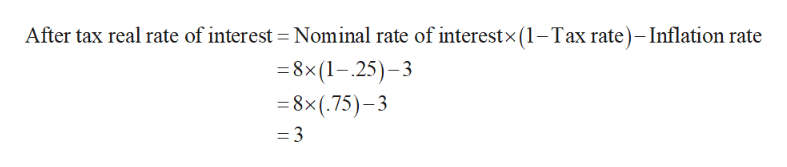# You put money into an account that earns a 8 percent nominal interest rate. The inflation rate is 3 percent, and your marginal tax rate is 25 percent. What is your after-tax real rate of interest? a.3 percent b.3.75 percent c.5 percent d.6 percent

Question

You put money into an account that earns a 8 percent nominal interest rate. The inflation rate is 3 percent, and your marginal tax rate is 25 percent. What is your after-tax real rate of interest?

 a. 3 percent b. 3.75 percent c. 5 percent d. 6 percent
check_circleExpert Solution
Step 1

The after- tax-real rate of interest is the actual financial benefit from an investment after considering all the effects of taxes and inflation.

Step 2

If the nominal rate of interest is 8%, inflation rate is 3%, marginal tax rate i...help_outlineImage TranscriptioncloseAfter tax real rate of interest Nominal rate of interestx (1-Tax rate)- Inflation rate 8x(1-25)-3 =8x(.75)-3 = 3 fullscreen

### Want to see the full answer?

See Solution

#### Want to see this answer and more?

Solutions are written by subject experts who are available 24/7. Questions are typically answered within 1 hour*

See Solution
*Response times may vary by subject and question
Tagged in